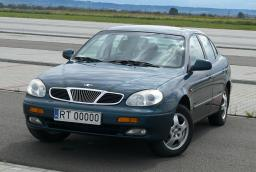# Freight and passenger car

The truck starts at 8 pm at 30 km/h. The passenger car starts at 8 pm at 40 km/h. Passenger arrives in the destination city 1 hour and 45 min earlier. What is the distance between the city of departure and the destination city?

s =  210 km

### Step-by-step explanation:

30 t = 40 (t-1.75)
s = 30 t

30·t = 40·(t-1.75)
s = 30·t

10t = 70
s-30t = 0

Pivot: Row 1 ↔ Row 2
s-30t = 0
10t = 70

t = 70/10 = 7
s = 0+30t = 0+30 · 7 = 210

s = 210
t = 7

Our linear equations calculator calculates it.Did you find an error or inaccuracy? Feel free to write us. Thank you!

Tips for related online calculators
Do you want to convert length units?
Do you want to convert velocity (speed) units?
Do you want to convert time units like minutes to seconds?